International
Tables for
Crystallography
Volume B
Reciprocal space
Edited by U. Shmueli

International Tables for Crystallography (2010). Vol. B, ch. 1.5, pp. 176-177   | 1 | 2 |

## Section 1.5.3.2. Space groups

M. I. Aroyoa* and H. Wondratschekb

aDepartamento de Fisíca de la Materia Condensada, Facultad de Cienca y Technología, Universidad del País Vasco, Apartado 644, 48080 Bilbao, Spain , and bInstitut für Kristallographie, Universität, D-76128 Karlsruhe, Germany
Correspondence e-mail:  wmpararm@lg.ehu.es

#### 1.5.3.2. Space groups

| top | pdf |

In crystallography one deals with real crystals. In many cases the treatment of the crystal is much simpler, but nevertheless describes the crystal and its properties very well, if the real crystal is replaced by an ideal crystal'. The real crystal is then considered to be a finite piece of an undisturbed, periodic, and thus infinitely extended arrangement of particles or their centres: ideal crystals are periodic objects in three-dimensional point space, also called direct space. Periodicity means that there are translations among the symmetry operations of ideal crystals. The symmetry group of an ideal crystal is called its space group.

Space groupsare of special interest for our problem because:

 (1) their irreps are the subject of the classification to be discussed; (2) this classification makes use of the isomorphism of certain groups to the so-called symmorphic space groups.

Therefore, space groups are introduced here in a slightly more detailed manner than the other concepts. In doing this we follow the definitions and symbolism of IT A, Part 8.

To each space groupbelongs an infinite setof translations, the translation subgroup of. The groupforms an infinite Abelian invariant subgroup of. For each translation its translation vector is defined. The set of all translation vectors is called the vector lattice L of. Because of the finite size of the atoms constituting the real crystal, the lengths of the translation vectors of the ideal crystal cannot be arbitrarily small; rather there is a lower limit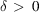for their length in the range of a few Å.

When referred to a coordinate system, consisting of an origin O and a basis, the symmetry operations, i.e. the elements: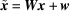of the space group, are described by matrix–column pairs (W, w) with matrix part W and column part w. The translations ofare represented by pairs, where I is theunit matrix andis the column of coefficients of the translation vector. The basis can always be chosen such that all columnsand no other columns of translations consist of integers. Such a basisis called a primitive basis. For each vector lattice L there exists an infinite number of primitive bases.

The space groupcan be decomposed into right cosets relative to: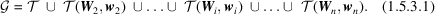The coset representatives form the finite set V =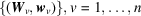, with, where I is the unit matrix and o is the column consisting of zeros only. The factor groupis isomorphic to the point groupof(called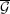in books on representation theory) describing the symmetry of the external shape of the macroscopic crystal and being represented by the matrices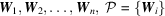. If V can be chosen such that all, thenis called a symmorphic space group. A symmorphic space group can be recognized easily from its conventional Hermann–Mauguin symbol which does not contain any screw or glide component. In terms of group theory, a symmorphic space group is the semidirect product ofand, cf. BC, p. 44. In symmorphic space groups(and in no others) there are site-symmetry groups which are isomorphic to the point groupof. (For the term site-symmetry group', see Section 1.5.4.2.)

Space groups can be classified into 219 (affine) space-group types either by isomorphism or by affine equivalence; the 230 crystallographic space-group types are obtained by restricting the transformations available for affine equivalence to those with positive determinant, cf. IT A, Section 8.2.1. Many important properties of space groups are shared by all space groups of a type. In such a case one speaks of properties of the type. For example, if a space group is symmorphic, then all space groups of its type are symmorphic, so that one normally speaks of a symmorphic space-group type.

With the concept of symmorphic space groups one can also define the arithmetic crystal classes: Letbe a symmorphic space group referred to a primitive basis andits set of coset representatives withfor all columns. Toall those space groupscan be assigned for which a primitive basis can be found such that the matrix partsof their sets V are the same as those of, only the columnsmay differ. In this way, to a type of symmorphic space groups, other types of space groups are assigned, i.e. the space-group types are classified according to the symmorphic space-group types. These classes are called arithmetic crystal classes of space groups or of space-group types.

There are 73 arithmetic crystal classes corresponding to the 73 types of symmorphic space groups; between 1 and 16 space-group types belong to an arithmetic crystal class. A matrix-algebraic definition of arithmetic crystal classes and a proposal for their nomenclature can be found in IT A, Section 8.2.3; see also Section 8.3.4and Table 8.3.4.1.